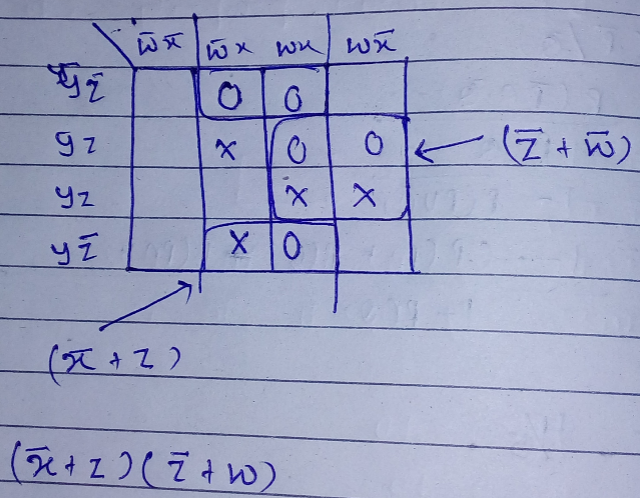Skip to content
Related Articles
GATE | GATE-CS-2017 (Set 2) | Question 63
• Last Updated : 16 Feb, 2017

Given f(w, x, y, z) = Σm(0,1,2,3,7,8,10) + Σd(5,6,11,15), where d represents the don’t-care condition in Karnaugh maps. Which of the following is a minimum product-of-sums(POS) form of f(w,x,y,z)?

(A) f = (w’ + z’ )( x’ + z )
(B) f = (w’ + z ) ( x + z )
(C) f = ( w + z ) ( x ‘ + z )
(D) f = ( w + z’ ) ( x’ + z )

Answer: (A)

Explanation:Quiz of this Question

Attention reader! Don’t stop learning now. Learn all GATE CS concepts with Free Live Classes on our youtube channel.

My Personal Notes arrow_drop_up